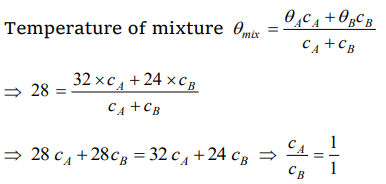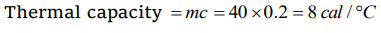## Thermal Properties of Matter Questions and Answers Part-9

1. Two liquids A and B are at 32°C and 24°C. When mixed in equal masses the temperature of the mixture is found to be 28°C. Their specific heats are in the ratio of
a) 3 : 2
b) 2 : 3
c) 1 : 1
d) 4 : 3

Explanation:2. A beaker contains 200 gm of water. The heat capacity of the beaker is equal to that of 20 gm of water. The initial temperature of water in the beaker is 20°C. If 440 gm of hot water at 92°C is poured in it, the final temperature (neglecting radiation loss) will be nearest to
a) 58°C
b) 68°C
c) 73°C
d) 78°C

Explanation:3. Amount of heat required to raise the temperature of a body through 1K is called its
a) Water equivalent
b) Thermal capacity
c) Entropy
d) Specific heat

Explanation:4. A metallic ball and highly stretched spring are made of the same material and have the same mass. They are heated so that they melt, the latent heat required
a) Are the same for both
b) Is greater for the ball
c) Is greater for the spring
d) For the two may or may not be the same depending upon the metal

Explanation: Latent heat is independent of configuration. Ordered energy spent in stretching the spring will not contribute to heat which is disordered kinetic energy of molecules of substance

5. A liquid of mass m and specific heat c is heated to a temperature 2T. Another liquid of mass m/2 and specific heat 2c is heated to a temperature T. If these two liquids are mixed, the resulting temperature of the mixture is
a) (2/3)T
b) (8/5)T
c) (3/5)T
d) (3/2)T

Explanation:6. Calorie is defined as the amount of heat required to raise temperature of 1g of water by 1°C and it is defined under which of the following conditions
a) From 14.5°C to 15.5°C at 760 mm of Hg
b) From 98.5°C to 99.5°C at 760 mm of Hg
c) From 13.5°C to 14.5°C at 76 mm of Hg
d) From 3.5°C to 4.5°C at 76 mm of Hg

Explanation: From 14.5°C to 15.5°C at 760 mm of Hg

7. 100 gm of ice at 0°C is mixed with 100 g of water at 100°C. What will be the final temperature of the mixture
a) 10°C
b) 20°C
c) 30°C
d) 40°C

Explanation:8. At atmospheric pressure, the water boils at 100°C. If pressure is reduced, it will boil at
a) Higher temperature
b) Lower temperature
c) At the same temperature
d) At critical temperature

Explanation: When pressure decreases, boiling point also decreases

9. A closed bottle containing water at 30°C is carried to the moon in a space-ship. If it is placed on the surface of the moon, what will happen to the water as soon as the lid is opened
a) Water will boil
b) Water will freeze
c) Nothing will happen on it
d) It will decompose into $H_{2}$ and $O_{2}$

10. The thermal capacity of 40 gm of aluminium (specific heat = $0.2cal\diagup gm\diagup° C$   )
a) 40 $cal\diagup° C$
b) 160 $cal\diagup° C$
c) 200 $cal\diagup° C$
d) 8 $cal\diagup° C$